AdaBoost算法

算法概述
算法描述

给定训练样本

(x1,y1),...(xi,yi)，...(xm,ym)
$\left ( x_{1} ,y_{1}\right ),...\left ( x_{i} ,y_{i}\right )，...\left ( x_{m} ,y_{m}\right )$ 其中

xi
$x_{i}$表示第

i
$i$个样本， 表示为负样本， 表示为正样本。m$m$为训练样本总数初始化训练样本的权重(

1m
$\frac{1}{m}$)第一次循环，首先训练一个弱分类器，计算该分类器的错误率；更改阈值使得错误率最低，更行样本权重经过T次循环，得到T个弱分类器，根据每个分类器正确分类的贡献作为权重进行加权组合，最后得到强分类器。

算法示意图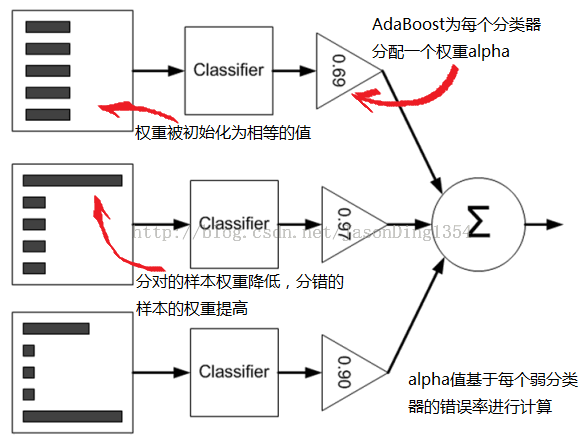算法流程
训练了T个弱分类器

ht,t∈{1,...,T}
$h_{t}, t\in \left \{ 1,..., T \right \}$。这些分类器很简单。大多数情况是只包含一次分裂的决策树。最后做决定的时候将赋值权重

αt
$\alpha_{t}$给每个分类器。输入特征向量为

xi
$x_{i}$，类别标签为

yi,i∈{1,..m}
$y_{i},i\in \left\{1,..m \right\}$，且

yi∈{1,−1}
$y_{i}\in\left\{1, -1 \right\}$。首先初始化样本全职

Dt(i)
$D_{t}\left(i\right)$来告诉分类器将一个数据点分类错误的代价是多少。

D1(i)=1/m,i=1,...,m
$D_{1}\left(i\right)=1/m, i=1,...,m$针对

t=1,...,T
$t=1,...,T$:  a. 寻找是的权重为

Dt(i)
$D_{t}\left(i\right)$的总错误最小的分类器

ht
$h_{t}$  b.求

ht=argminhj∈Hεj
$h_{t}=argmin_{h_{j}\in H}\varepsilon_{j}$，

εj=∑mi=1Dt(i)
$\varepsilon_{j}=\sum_{i=1}^mD_{t}\left(i\right)$(其中

yi≠hj(xi)
$y_{i}\neq h_{j}\left(x_{i}\right)$)，如果最小错误满足

ε<0.5
$\varepsilon<0.5$则继续；否则退出  c. 设置

ht
$h_{t}$的权重

αt=log[(1−εt)/εt]
$\alpha_{t}=log[\left(1-\varepsilon_{t} \right)/\varepsilon_{t}]$，这儿

εt
$\varepsilon_{t}$为步骤2b中的最小错误  d. 更新数据点权重:

Dt+1(i)=[Dt(i)e(−αtyiht(xi))]/Zt
$D_{t+1}\left(i\right) = [D_{t}\left(i\right)e^{\left(-\alpha_{t}y_{i}h_{t}\left(x_{i}\right)\right)}]/Z_{t}$，这

Zt
$Z_{t}$将所有数据点权重归一化算法结束后，最后的强分类器几首输入向量

x
$x$，使用所有弱分类器的加权和进行分类
H(x)=sign(∑t=1Tαtht(x))H\left(x\right)=sign\left(\sum_{t=1}^T\alpha_{t}h_{t}\left(x\right)\right)

示例代码
'''
Created on Dec 26th
@author: zfluo
'''
from numpy import *
datMat = matrix([[1., 2.1],
[2., 1.1],
[1.3, 1.],
[1., 1.],
[2., 1.]])
classLabels = [1.0, 1.0, -1.0, -1.0, -1.0]
return datMat, classLabels

# 构建单层决策树， lt: less than, gt: greater than
def stumpClassify(dataMatrix, dimen, threshVal, threshIneq):
retArray = ones((shape(dataMatrix), 1))
if threshIneq == 'lt':
retArray[dataMatrix[:, dimen] <= threshVal] = -1.0
else:
retArray[dataMatrix[:, dimen] > threshVal] = -1.0
return retArray

# 寻找最优分支
def buildStump(dataArr, classLabels, D):
dataMatrix = mat(dataArr)
labelMat = mat(classLabels).T
m, n = shape(dataMatrix)
numSteps = 10.0; bestStump = {}; bestClasEst = mat(zeros((m, 1)))
minError = inf
for i in range(n):
rangeMin = dataMatrix[:, i].min()
rangeMax = dataMatrix[:, i].max()
stepSize = (rangeMax - rangeMin)/numSteps
for j in range(-1, int(numSteps) + 1):
for inequal in ['lt', 'gt']:
threshVal = (rangeMin + float(j)*stepSize)
predictedVals = stumpClassify(dataMatrix, i, threshVal, inequal)
errArr = mat(ones((m, 1)))
errArr[predictedVals == labelMat] = 0
weightedError = D.T*errArr
# print('split: dim %d, thresh %.2f, thresh ineqal: %s, the weighted error is %.3f' %
#       (i, threshVal, inequal, weightedError))
if weightedError < minError:
minError = weightedError
bestClasEst = predictedVals.copy()
bestStump['dim'] = i
bestStump['thresh'] = threshVal
bestStump['ineq'] = inequal
return bestStump, minError, bestClasEst

def adaBoostTrainDS(dataArr, classLabels, numIt = 40):
weakClassArr = []
m = shape(dataArr)
D = mat(ones((m, 1))/m)
aggClassEst = mat(zeros((m,1)))
for i in range(numIt):
bestStump, error, classEst = buildStump(dataArr, classLabels, D)
print('D:', D.T)
alpha = float(0.5*log((1.0 - error)/max(error, 1e-16)))
bestStump['alpha'] = alpha
weakClassArr.append(bestStump)
print('classEst: ', classEst.T)
expon = multiply(-1*alpha*mat(classLabels).T, classEst)
D = multiply(D, exp(expon))
D = D/D.sum()
aggClassEst += alpha*classEst
print('aggClassEst: ', aggClassEst.T)
aggErrors = multiply(sign(aggClassEst)!= mat(classLabels).T, ones((m, 1)))
errorRate = aggErrors.sum()/m
print('total error: ', errorRate, '\n')
if errorRate == 0.0:
break
return weakClassArr, aggClassEst

dataMatrix = mat(datToClass)
m = shape(dataMatrix)
aggClassEst = mat(zeros((m, 1)))
for i in range(len(classifierArr)):
classEst = stumpClassify(dataMatrix, classifierArr[i]['dim'], \
classifierArr[i]['thresh'],  \
classifierArr[i]['ineq'])
aggClassEst += classifierArr[i]['alpha']*classEst
print(aggClassEst)
return sign(aggClassEst)

dataMat = []; labelMat = []
fr = open(fileName)
lineArr = []
curLine = line.strip().split('\t')
for i in range(numFeat - 1):
lineArr.append(float(curLine[i]))
dataMat.append(lineArr)
labelMat.append(float(curLine[-1]))
return dataMat, labelMat

# 绘制ROC曲线及AUC计算函数
def plotROC(preStrengths, classLabels):
import matplotlib.pyplot as plt
cur = (1.0, 1.0)
ySum = 0.0
numPosClas = sum(array(classLabels) == 1.0)
yStep = 1/float(numPosClas)
xStep = 1/float(len(classLabels) - numPosClas)
sortedIndicies = preStrengths.argsort()
fig = plt.figure()
fig.clf()
ax = plt.subplot(111)
for index in sortedIndicies.tolist():
if classLabels[index] == 1.0:
delX = 0; delY = yStep
else:
delX = xStep; delY = 0
ySum += cur
ax.plot([cur, cur - delX], [cur, cur - delY], c = 'b')
cur = (cur - delX, cur - delY)
ax.plot([0, 1], [0, 1], 'b--')
plt.xlabel('False Positive Rate')
plt.ylabel('True Positive Rate')
ax.axis([0, 1, 0, 1])
plt.show()
print('the Area Under the Curve is:', ySum*xStep)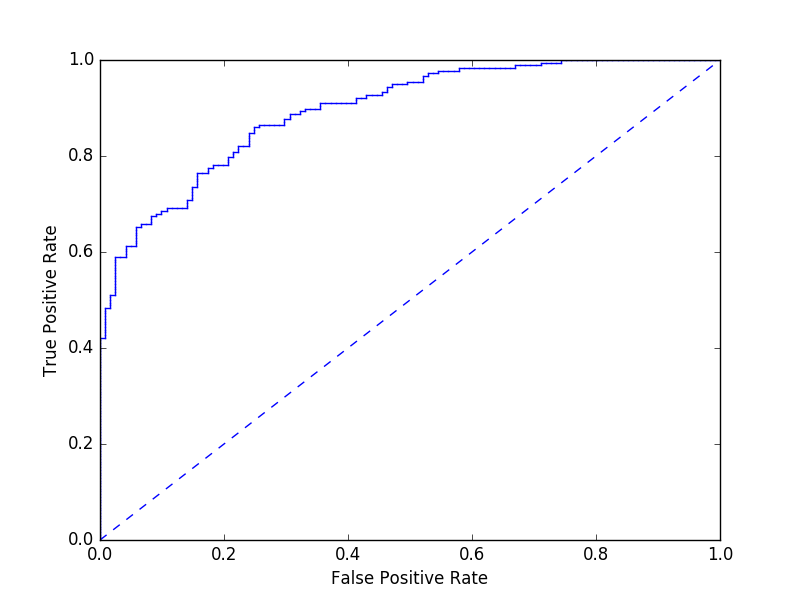算法特点

优点： 泛化错误率低，易编码，适用于大部分分类器，误参数调整  缺点： 对离群点敏感  使用数据类型：数值型和标称型

展开全文文章目录
小结Boosting算法回顾Boosting算法的流程是：首先训练处一个弱学习器，根据弱学习器的误差率更新训练样本的权重，然后基于调整权重后的训练集训练第二个弱学习器，直到弱学习器达到事先指定的数目T，停止算法。
对于Boosting算法的流程，可以看到如果我们解决以下4个问题，既可以得到完整的Boosting算法
弱学习器的误差率训练样本的权重

w

w

更新方法更新样本权重的方法结合策略

f

(

x

)

=

∑

k

=

1

K

α

k

G

k

(

x

)

f(x) = \sum_{k=1}^K \alpha_kG_k(x)

其中

K

K

是

K

K

个弱学习器。

k

−

1

k-1

次迭代后，第

k

−

1

k-1

轮后强学习器为 KaTeX parse error: No such environment: align at position 8: \begin{̲a̲l̲i̲g̲n̲}̲ f_{k-1}(x) & =… 经过

k

k

次迭代后，第

k

k

轮后强学习器为

f

k

(

x

)

=

∑

i

=

1

k

α

i

G

i

(

x

)

=

f

k

−

1

(

x

)

+

α

k

G

k

(

x

)

f_k(x) = \sum_{i=1}^k \alpha_i G_i(x) = f_{k-1}(x) + \alpha_kG_k(x)

得到第

k

k

α

k

\alpha_k

和

G

k

(

x

)

G_k(x)

使

f

k

(

x

)

f_k(x)

在训练数据集上的指数损失最小，即AdaBoost的目标函数为 KaTeX parse error: No such environment: align at position 8: \begin{̲a̲l̲i̲g̲n̲}̲ (\alpha_k,G_k(…   由于

e

[

−

y

i

(

f

k

−

1

(

x

i

)

)

]

e^{[{-y_i(f_{k-1}(x_i))}]}

的值不依赖

α

,

G

\alpha,G

，因此他与最小化无关，它仅仅依赖于随着每一轮迭代而变化的

f

k

−

1

(

x

)

f_{k-1}(x)

，因此可以把

e

[

−

y

i

(

f

k

−

1

(

x

i

)

)

]

e^{[{-y_i(f_{k-1}(x_i))}]}

看做

w

‾

k

i

\overline{w}_{ki}

，即目标函数变为

(

α

k

,

G

k

(

x

)

)

=

arg

⁡

m

i

n

⏟

α

,

G

∑

i

=

1

m

w

‾

k

i

e

[

−

y

i

(

α

G

(

x

i

)

)

]

(\alpha_k,G_k(x)) = \underbrace{\arg\,min}_{\alpha,G}\sum_{i=1}^m \overline{w}_{ki} e^{[{-y_i(\alpha{G(x_i)}})]}

α

k

∗

\alpha_k^*

和

G

k

∗

(

x

)

G_k^*(x)

。
首先，对于任意的

α

>

0

\alpha>0

，

G

k

∗

(

x

)

G_k^*(x)

表示第

k

k

轮能够使得加训练数据分类误差率最小的基本分类器，分类误差率为

e

k

=

∑

i

=

1

m

w

‾

k

i

I

(

y

i

≠

G

k

(

x

i

)

)

∑

i

=

1

m

w

‾

k

i

=

∑

i

=

1

m

w

‾

k

i

I

(

y

i

≠

G

k

(

x

i

)

)

=

∑

y

i

≠

G

k

(

x

i

)

w

‾

k

i

e_k = {\frac{\sum_{i=1}^m\overline{w}_{ki}I(y_i\neq{G_k(x_i)})}{\sum_{i=1}^m\overline{w}_{ki}}} = \sum_{i=1}^m\overline{w}_{ki}I(y_i\neq{G_k(x_i)}) = \sum_{{y_i}\neq{G_k(x_i)}}\overline{w}_{ki}

G

k

∗

(

x

)

G_k^*(x)

为

G

k

∗

(

x

)

=

a

r

g

min

⁡

⏟

G

∑

i

=

1

m

w

‾

k

i

I

(

y

i

≠

G

(

x

i

)

)

G_k^*(x) = \underbrace{arg\,\min}_{G}\sum_{i=1}^m \overline{w}_{ki} I(y_i\neq{G(x_i))}

G

k

∗

(

x

)

G_k^*(x)

即为学习器的

G

k

(

x

)

G_k(x)

，把

G

k

(

x

)

G_k(x)

代入目标函数对

α

\alpha

求导并使导数为0，可以把上述的目标函数优化成 KaTeX parse error: No such environment: align at position 8: \begin{̲a̲l̲i̲g̲n̲}̲ (\alpha_k,G_k(… 既得最小的

α

\alpha

为

α

k

∗

=

1

2

log

⁡

1

−

e

k

e

k

\alpha_k^* = {\frac{1}{2}}\log{\frac{1-e_k}{e_k}}

最后看样本的权重更新，利用

f

k

(

x

)

=

f

k

−

1

(

x

)

+

α

k

G

k

(

x

)

f_k(x)=f_{k-1}(x)+\alpha_kG_k(x)

和

w

‾

k

i

=

e

[

−

y

i

f

k

−

1

(

x

i

)

]

\overline{w}_{ki}=e^{[-y_if_{k-1}(x_i)]}

可得 KaTeX parse error: No such environment: align at position 8: \begin{̲a̲l̲i̲g̲n̲}̲ \overline{w}_{…

w

‾

k

+

1

,

i

\overline{w}_{k+1,i}AdaBoost算法既可以解决分类问题，又可以解决回归问题。对于分类问题，此处我们讲述的AdaBoost算法流程主要是针对二分类问题，二分类问题和多分类问题的区别主要在于弱分类器的系数上，本文会介绍AdaBoost SAMME算法如何计算弱分类器的系数；对于回归问题，由于AdaBoost用来解决回归问题的变种有很多，本文只对AdaBoost R2算法做一个介绍。
输入

m

m

个样本

n

n

个特征的训练数据集

T

=

{

(

x

1

,

y

1

)

,

(

x

2

,

y

2

)

,

⋯

,

(

x

m

,

y

m

)

}

T=\{(x_1,y_1),(x_2,y_2),\cdots,(x_m,y_m)\}

。
针对二分类问题，

y

i

∈

Y

=

{

1

,

−

1

}

y_i\in{Y=\{1,-1\}}

。
输出
最终强学习器

G

(

x

)

G(x)

。
强分类器流程
初始化训练数据的权重

D

1

=

(

w

11

,

⋯

,

w

1

i

,

⋯

,

w

1

m

)

,

w

1

i

=

1

m

,

i

=

1

,

2

,

⋯

,

m

生成弱分类器

G

k

(

x

)

,

k

=

1

,

2

,

⋯

,

K

计算弱分类器

G

k

(

x

)

G_k(x)

在训练集上的分类误差率为 KaTeX parse error: No such environment: align at position 8: \begin{̲a̲l̲i̲g̲n̲}̲ e_k & = \sum_{…计算

G

k

(

x

)

G_k(x)

的权重系数 KaTeX parse error: No such environment: align at position 8: \begin{̲a̲l̲i̲g̲n̲}̲ & \alpha_k={\f…   二分类问题的权重系数中，可以看出如果分类误差率

e

k

e_k

越大，则对应的弱分类器的权重系数

α

k

\alpha_k

越小，即误差率小的弱分类器权重系数越大。

R

R

为类别数，如果

R

=

2

R=2

，则该多元分类的权重系数将变成二元分类的权重系数。 5. 更新训练数据的权重 KaTeX parse error: No such environment: align at position 8: \begin{̲a̲l̲i̲g̲n̲}̲ & D_{k+1} = (w…   其中

Z

k

Z_k

是规范因子

Z

k

=

∑

i

=

1

m

w

k

i

e

−

α

k

y

i

G

k

(

x

i

)

Z_k=\sum_{i=1}^mw_{ki}e^{-\alpha_ky_iG_k(x_i)}

从

w

k

+

1

,

i

w_{k+1,i}

的计算公式中可以看出，如果第

i

i

个样本分类错误，则

y

i

G

k

(

x

i

)

<

0

y_iG_k(x_i)<0

，导致样本的权重在第

k

+

1

k+1

个弱分类器中变大，反之，则样本权重在第

k

+

1

k+1

个弱分类器中变小。 6. 结合策略 KaTeX parse error: No such environment: align at position 8: \begin{̲a̲l̲i̲g̲n̲}̲ & f(x)=\sum_{k…
强回归器流程
初始化训练数据的权重

D

1

=

(

w

11

,

⋯

,

w

1

i

,

⋯

,

w

1

m

)

,

w

1

i

=

1

m

,

i

=

1

,

2

,

⋯

,

m

生成弱分类器

G

k

(

x

)

,

k

=

1

,

2

,

⋯

,

K

计算弱回归器

G

k

(

x

)

G_k(x)

在训练集上的最大误差

E

k

=

max

⁡

∣

y

i

−

G

k

(

x

i

)

∣

,

i

=

1

,

2

,

⋯

,

m

计算每个样本之间的相对误差

e

k

i

=

∣

y

i

−

G

k

(

x

i

)

∣

E

k

e_{ki}={\frac{|y_i-G_k(x_i)|}{E_k}}

此处也可以使用均方误差，即

e

k

i

=

(

y

i

−

G

k

(

x

i

)

)

2

E

k

2

e_{ki}={\frac{(y_i-G_k(x_i))^2}{E_k^2}}

计算第

k

k

弱回归器的误差率和权重系数 KaTeX parse error: No such environment: align at position 8: \begin{̲a̲l̲i̲g̲n̲}̲ & e_k = \sum_{…更新训练数据的权重

w

k

+

1

,

i

=

w

k

i

Z

k

α

k

1

−

e

k

i

w_{k+1,i} = {\frac{w_{ki}}{Z_k}\alpha_k^{1-e_{ki}}}

其中

Z

k

Z_k

是规范因子

Z

k

=

∑

i

=

1

m

w

k

i

α

k

1

−

e

k

i

Z_k = \sum_{i=1}^m w_{ki}\alpha_k^{1-e_{ki}}

结合策略

G

(

x

)

=

G

k

∗

(

x

)

G(x) = G_{k^*}(x)

其中

G

k

∗

(

x

)

G_{k^*}(x)

是所有

ln

⁡

1

α

k

,

k

=

1

,

2

,

⋯

,

K

的中位数对应序号

k

∗

k^*

对应的弱回归器
优点
不容易过拟合分类精准度高由于弱分类器既可以是分类器又可以是回归器，使用灵活
缺点
由于是对训练数据加权，有可能会赋予训练数据中的异常值较高的权重，影响模型的准确度
小结展开全文AdaBoost 算法 是一种经典的集成学习算法，它将多个弱分类器集成起来，以达到较高的分类准确率，广泛应用于数据分类、人脸检测等应用中。尤其在人脸检测方面，AdaBoost 是非常经典、成功的一个算法。弱分类器被线性组合成为一个强分类器。
一、面临两个问题：
在每一轮，如何改变训练数据的概率分布或者权值分布。如何将弱分类器组合成强分类器。
提高那些被前一轮弱分类器错误分类样本的权值，降低那些被正确分类的样本的权值。采用加权多数表决。具体的，加大分类错误率低的分类器的权值，使其在表决中起较大作用，减少分类误差率大的弱分类器的权值，使其在表决中起较小作用。
三、训练目标：
最小化指数损失函数。
四、三部分组成：
初始化训练数据的权值分布。如果有N个样本，则每一个训练样本最开始时都被赋予相同的权值：1/N。训练弱分类器。具体训练过程中，如果某个样本点已经被准确地分类，那么在构造下一个训练集中，它的权值就被降低；相反，如果某个样本点没有被准确地分类，那么它的权值就得到提高。然后，权值更新过的样本集被用于训练下一个分类器，整个训练过程如此迭代地进行下去。将各个训练得到的弱分类器组合成强分类器。各个弱分类器的训练过程结束后，加大分类误差率小的弱分类器的权重，使其在最终的分类函数中起着较大的决定作用，而降低分类误差率大的弱分类器的权重，使其在最终的分类函数中起着较小的决定作用。换言之，误差率低的弱分类器在最终分类器中占的权重较大，否则较小。
五、具体步骤：
输人参数：e是预测误差，m 是需要构建的单决策器的个数，如：
if(x < 3 )
y ﹦1
else
y =-1
就是一个简单的单决策器，基于x 预测y。α是每个单决策器的不同权重。n 是数据集中点的个数。
W = [ 1/n，1/n，…，1/n ]   # W是一个数组，表示每个数据点的权重，初始相同
C = {};  # C 是一个集合，存放每次循环得到的最佳单决策器
For i = 1 : m
1) 在当前的权重W 下，尝试各种不同的单决策器，直到找到预测误差最小的那个单决策器Hi(针对所有数据),使得误分的数据点的权重之和最小。对Hi预测错误的数据点，计算这些点的权重之和εi；
2) C ﹦C 与 Hi 的并集；
3) 计算分类器的权重αi, αi = 1/2 *log((1-εi + /εi) ；
4) 更新W 数组，
w_right = exp( - αi) ，w_wrong = exp( αi) ； # exp 是自然对数
预测正确的数据点，原始权重乘以w_right；
预测错误的数据点，原始权重乘以w_rong；
5) W = W/sum(W) ;    # 规范化W，规范化之后，使各元素之和为1
6) 对C 中保留的所有分类器，集成决策器为：H_all = α1 * H1 + …αi* Hi
7)使用H_all对所有数据点再次预测，如果 H_a11 预测的误差e为0 或小于某个阈值，终止 for 循环；否则继续 for 循环。
模式识别、计算机视觉领域，用于二分类和多分类场景
参考：
《数据挖掘必备算法详解》https://blog.csdn.net/u012258999/article/details/42457577
展开全文机器学习


算法分析　  　该算法其实是一个简单的弱分类算法提升过程，这个过程通过不断的训练，可以提高对数据的分类能   Adaboost 力。整个过程如下所示： 　　         1. 先通过对N个训练样本的学习得到第一个弱分类器； 　　         2. 将分错的样本和其他的新数据一起构成一个新的N个的训练样本，通过对这个样本的学习得到第二个弱分类器 ； 　　        3. 将1和2都分错了的样本加上其他的新样本构成另一个新的N个的训练样本，通过对这个样本的学习得到第三个弱分类器；  　　4. 最终经过提升的强分类器 。即某个数据被分为哪一类要通过 ， ……的多数表决。
初始化训练数据的权值分布。如果有N个样本，则每一个训练样本最开始时都被赋予相同的权重：1/N。 训练弱分类器。具体训练过程中，如果某个样本点已经被准确地分类，那么在构造下一个训练集中，它的权重就被降低；相反，如果某个样本点没有被准确地分类，那么它的权重就得到提高。然后，权重更新过的样本集被用于训练下一个分类器，整个训练过程如此迭代地进行下去。将各个训练得到的弱分类器组合成强分类器。各个弱分类器的训练过程结束后，加大分类误差率小的弱分类器的权重，使其在最终的分类函数中起着较大的决定作用，而降低分类误差率大的弱分类器的权重，使其在最终的分类函数中起着较小的决定作用。换言之，误差率低的弱分类器在最终分类器中占的权重较大，否则较小。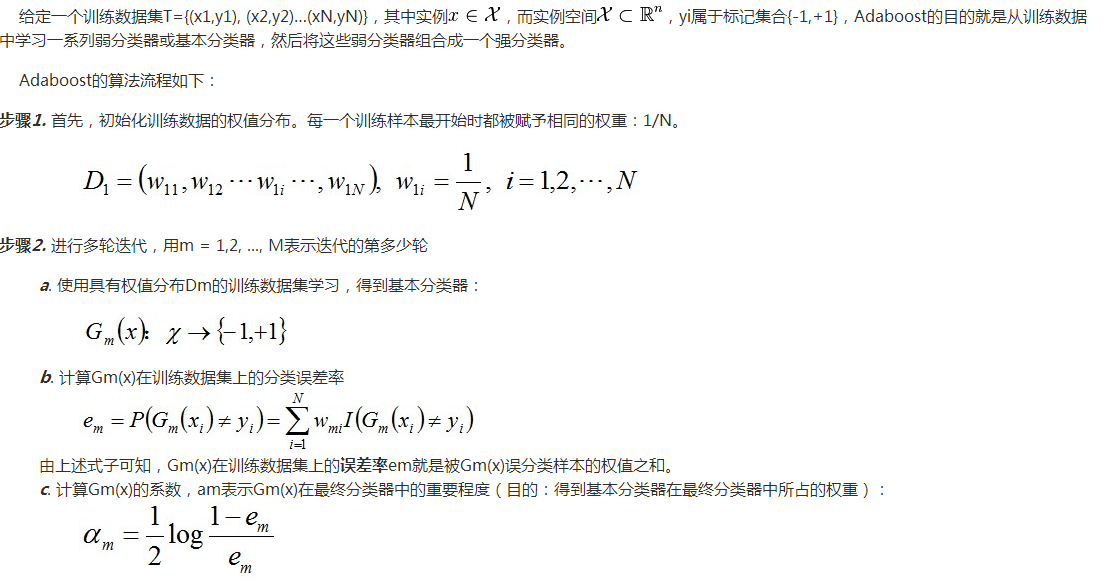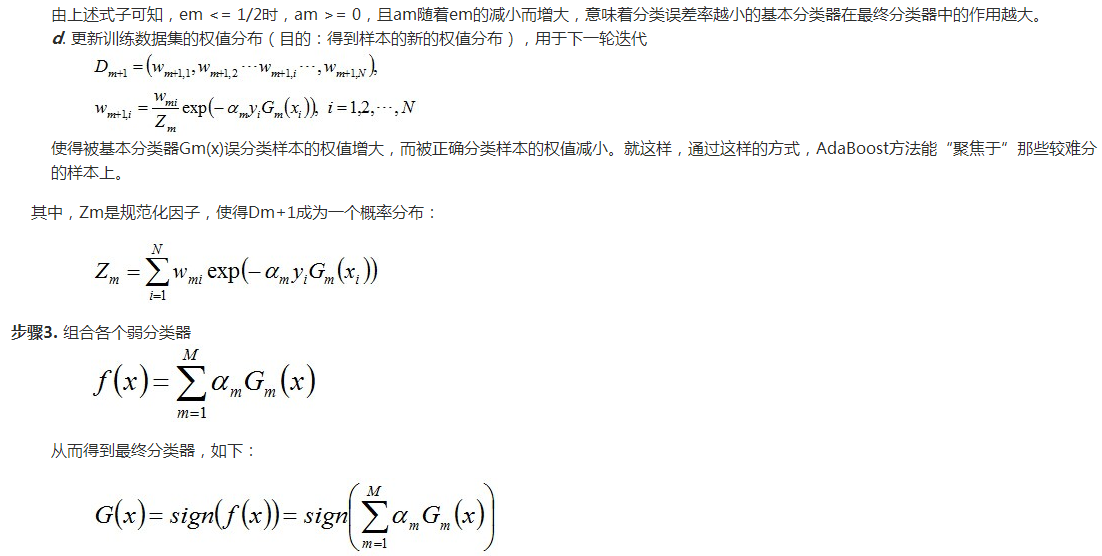对于这个算法需要介绍的是：  1.         算法开始前，需要将每个样本的权重初始化为1/m,这样一开始每个样本都是等概率的分布，每个分类器都会公正对待。  2.         开始迭代后，需要计算每个弱分类器的分类错误的误差，误差等于各个分错样本的权重和，这里就体现了样本权重的作用。如果一个分类器正确分类了一个权重大的样本，那么这个分类器的误差就会小，否则就会大。这样就对分类错误的样本更大的关注。  3.         获取最优分类器后，需要计算这个分类器的权重，然后再更新各个样本的权重，然后再归一化  4.         算法迭代的次数一般不超过弱分类器的个数，如果弱分类器的个数非常之多，那么可以权衡自己性价比来折中选择。  5.         迭代完成后，最后的分类器是由迭代过程中选择的弱分类器线性加权得到的。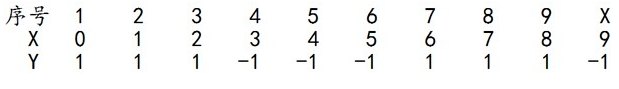求解过程：初始化训练数据的权值分布，令每个权值W1i = 1/N = 0.1，其中，N = 10，i = 1,2, ..., 10，然后分别对于m = 1,2,3, ...等值进行迭代。
拿到这10个数据的训练样本后，根据 X 和 Y 的对应关系，要把这10个数据分为两类，一类是“1”，一类是“-1”，根据数据的特点发现：“0 1 2”这3个数据对应的类是“1”，“3 4 5”这3个数据对应的类是“-1”，“6 7 8”这3个数据对应的类是“1”，9是比较孤独的，对应类“-1”。抛开孤独的9不讲，“0 1 2”、“3 4 5”、“6 7 8”这是3类不同的数据，分别对应的类是1、-1、1，直观上推测可知，可以找到对应的数据分界点，比如2.5、5.5、8.5 将那几类数据分成两类。当然，这只是主观臆测，下面实际计算下这个过程。
迭代过程1
对于m=1，在权值分布为D1（10个数据，每个数据的权值皆初始化为0.1）的训练数据上，经过计算可得：
阈值v取2.5时误差率为0.3（x < 2.5时取1，x > 2.5时取-1，则6 7 8分错，误差率为0.3），阈值v取5.5时误差率最低为0.4（x < 5.5时取1，x > 5.5时取-1，则3 4 5 6 7 8皆分错，误差率0.6大于0.5，不可取。故令x > 5.5时取1，x < 5.5时取-1，则0 1 2 9分错，误差率为0.4），阈值v取8.5时误差率为0.3（x < 8.5时取1，x > 8.5时取-1，则3 4 5分错，误差率为0.3）。
所以无论阈值v取2.5，还是8.5，总得分错3个样本，故可任取其中任意一个如2.5，弄成第一个基本分类器为：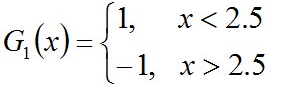上面说阈值v取2.5时则6 7 8分错，所以误差率为0.3，更加详细的解释是：因为样本集中
0 1 2对应的类（Y）是1，因它们本身都小于2.5，所以被G1(x)分在了相应的类“1”中，分对了。3 4 5本身对应的类（Y）是-1，因它们本身都大于2.5，所以被G1(x)分在了相应的类“-1”中，分对了。但6 7 8本身对应类（Y）是1，却因它们本身大于2.5而被G1(x)分在了类"-1"中，所以这3个样本被分错了。9本身对应的类（Y）是-1，因它本身大于2.5，所以被G1(x)分在了相应的类“-1”中，分对了。
从而得到G1(x)在训练数据集上的误差率（被G1(x)误分类样本“6 7 8”的权值之和）e1=P(G1(xi)≠yi) = 3*0.1 = 0.3。
然后根据误差率e1计算G1的系数：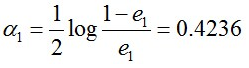这个a1代表G1(x)在最终的分类函数中所占的权重，为0.4236。 接着更新训练数据的权值分布，用于下一轮迭代：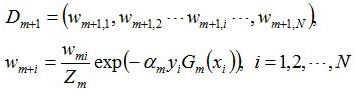值得一提的是，由权值更新的公式可知，每个样本的新权值是变大还是变小，取决于它是被分错还是被分正确。
即如果某个样本被分错了，则yi * Gm(xi)为负，负负等正，结果使得整个式子变大（样本权值变大），否则变小。
第一轮迭代后，最后得到各个数据新的权值分布D2 = (0.0715, 0.0715, 0.0715, 0.0715, 0.0715,  0.0715, 0.1666, 0.1666, 0.1666, 0.0715)。由此可以看出，因为样本中是数据“6 7 8”被G1(x)分错了，所以它们的权值由之前的0.1增大到0.1666，反之，其它数据皆被分正确，所以它们的权值皆由之前的0.1减小到0.0715。
分类函数f1(x)= a1*G1(x) = 0.4236G1(x)。
此时，得到的第一个基本分类器sign(f1(x))在训练数据集上有3个误分类点（即6 7 8）。
从上述第一轮的整个迭代过程可以看出：被误分类样本的权值之和影响误差率，误差率影响基本分类器在最终分类器中所占的权重。
迭代过程2
对于m=2，在权值分布为D2 = (0.0715, 0.0715, 0.0715, 0.0715, 0.0715,  0.0715, 0.1666, 0.1666, 0.1666, 0.0715)的训练数据上，经过计算可得：
阈值v取2.5时误差率为0.1666*3（x < 2.5时取1，x > 2.5时取-1，则6 7 8分错，误差率为0.1666*3），阈值v取5.5时误差率最低为0.0715*4（x > 5.5时取1，x < 5.5时取-1，则0 1 2 9分错，误差率为0.0715*3 + 0.0715），阈值v取8.5时误差率为0.0715*3（x < 8.5时取1，x > 8.5时取-1，则3 4 5分错，误差率为0.0715*3）。
所以，阈值v取8.5时误差率最低，故第二个基本分类器为：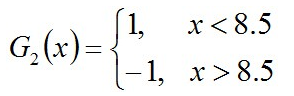面对的还是下述样本：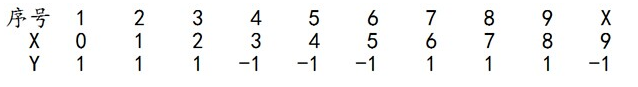很明显，G2(x)把样本“3 4 5”分错了，根据D2可知它们的权值为0.0715, 0.0715,  0.0715，所以G2(x)在训练数据集上的误差率e2=P(G2(xi)≠yi) = 0.0715 * 3 = 0.2143。
计算G2的系数：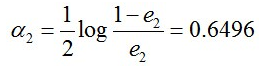更新训练数据的权值分布：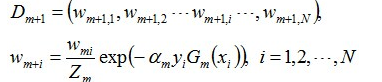D3 = (0.0455, 0.0455, 0.0455, 0.1667, 0.1667,  0.01667, 0.1060, 0.1060, 0.1060, 0.0455)。被分错的样本“3 4 5”的权值变大，其它被分对的样本的权值变小。 f2(x)=0.4236G1(x) + 0.6496G2(x)
此时，得到的第二个基本分类器sign(f2(x))在训练数据集上有3个误分类点（即3 4 5）。
迭代过程3
对于m=3，在权值分布为D3 = (0.0455, 0.0455, 0.0455, 0.1667, 0.1667,  0.01667, 0.1060, 0.1060, 0.1060, 0.0455)的训练数据上，经过计算可得：
阈值v取2.5时误差率为0.1060*3（x < 2.5时取1，x > 2.5时取-1，则6 7 8分错，误差率为0.1060*3），阈值v取5.5时误差率最低为0.0455*4（x > 5.5时取1，x < 5.5时取-1，则0 1 2 9分错，误差率为0.0455*3 + 0.0715），阈值v取8.5时误差率为0.1667*3（x < 8.5时取1，x > 8.5时取-1，则3 4 5分错，误差率为0.1667*3）。
所以阈值v取5.5时误差率最低，故第三个基本分类器为（下图画反了，待后续修正）：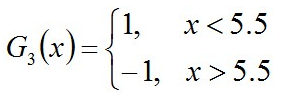依然还是原样本：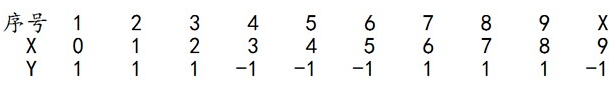此时，被误分类的样本是：0 1 2 9，这4个样本所对应的权值皆为0.0455，
所以G3(x)在训练数据集上的误差率e3= P(G3(xi)≠yi) =0.0455*4 = 0.1820。
计算G3的系数：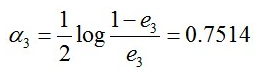更新训练数据的权值分布：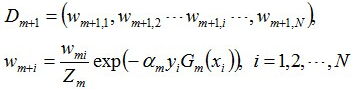D4 = (0.125, 0.125, 0.125, 0.102, 0.102,  0.102, 0.065, 0.065, 0.065, 0.125)。被分错的样本“0 1 2 9”的权值变大，其它被分对的样本的权值变小。
f3(x)=0.4236G1(x) + 0.6496G2(x)+0.7514G3(x)
此时，得到的第三个基本分类器sign(f3(x))在训练数据集上有0个误分类点。至此，整个训练过程结束。
G(x) = sign[f3(x)] = sign[ a1 * G1(x) + a2 * G2(x) + a3 * G3(x) ]，将上面计算得到的a1、a2、a3各值代入G(x)中，得到最终的分类器为：G(x) = sign[f3(x)] = sign[ 0.4236G1(x) + 0.6496G2(x)+0.7514G3(x) ]。
图文解释可以参考：
http://baidutech.blog.51cto.com/4114344/743809/
http://blog.csdn.net/haidao2009/article/details/7514787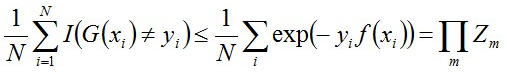下面，咱们来通过推导来证明下上述式子。
当G(xi)≠yi时，yi*f(xi)<0，因而exp(-yi*f(xi))≥1，因此前半部分得证。
关于后半部分，别忘了：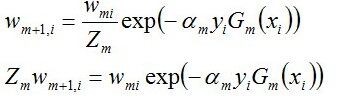整个的推导过程如下：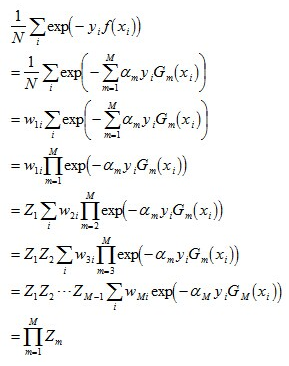这个结果说明，可以在每一轮选取适当的Gm使得Zm最小，从而使训练误差下降最快。接着，咱们来继续求上述结果的上界。
对于二分类而言，有如下结果：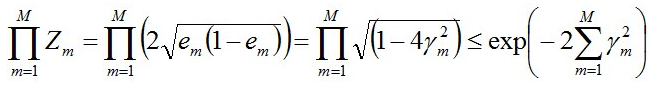其中，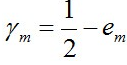。
继续证明下这个结论。
由之前Zm的定义式跟本节最开始得到的结论可知：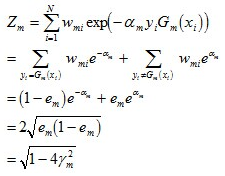而这个不等式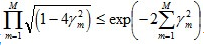可先由e^x和1-x的开根号，在点x的泰勒展开式推出。
值得一提的是，如果取γ1, γ2… 的最小值，记做γ（显然，γ≥γi>0，i=1,2,...m），则对于所有m，有：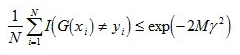参考：http://www.360doc.com/content/14/1109/12/20290918_423780183.shtml
3 模型的优缺点

转自：http://blog.csdn.net/tiandijun/article/details/48036025
展开全文python 机器学习adaboost源码
• 目录boost算法问题描述Adaboost 算法基本思路传送门 boost算法问题描述 1 如何计算学习误差率e 2 如何得到弱学习器权重系数α 3 如何更新样本权重D 4 使用何种结合策略 ...集成学习之Adaboost算法原理小结 ...
• 文章目录1 Adaboost算法简介2 集成学习2.1 集成学习概念2.2 bagging2.3 boosting2.4 bagging与boosting的区别2.5 组合策略(1) 平均法(2) 投票法(3) 学习法3 Adaboost 算法4 实验实验1 基于单层决策树构建弱分类器...分类 集成学习python 机器学习 算法 集成学习决策树 python
• 1. 集成学习（ensemble learing）背景介绍 集成学习（ensemble learing）通过构建并结合多个学习器（learner）来完成学习任务，通常...另一类是以boosting、Adaboost算法为代表的，个体学习器是串行序列化生成的...集成学习 boosting
• AdaBoost的前身和今世 强可学习和弱可学习 在概率近似正确(PAC)学习框架中, 一个类如果存在: 一个多项式复杂度的学习算法,正确率略大于随机猜测(例如二分类问题中大于1/2),称弱可学习的 一个多项式复杂度的学习...人工智能机器学习 提升
•  接自https://blog.csdn.net/Y_hero/article/details/88381259 ,由上一篇总结我们已经初步了解了集成学习以及Boosting的基本原理，不过有几个Boosting具体的问题没有详细...只要是boosting大家族的算法，都要解决这...机器学习数学建模 机器学习 python...